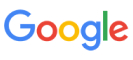New update is available. Click here to update.
Topics

# A Number Game

Moderate0/80
0 upvote## Problem statement

Ninja has an integer 'A'.

He will go through all integers 'X' from 1 to 10^18 and for every 'X', he will store the value of 'LCM(X,A) ÷ X'.

He wants to know the number of unique values he will obtain in the end.

Example :
``````‘A’ = '2'
For X = 1, Ninja will get LCM(1,2) ÷ 1 = 2/1 = 2.
For X = 2, Ninja will get LCM (2,2) ÷ 2 = 2/2 = 1.
For X = 3, Ninja will get LCM (3,2) ÷ 3  = 6/3 = 2.
For X = 4, Ninja will get LCM (4,2) ÷ 4 = 4/2 = 2.
For X = 5, Ninja will get LCM (5,2) ÷ 5 = 10/2 = 2.
For X = 6, Ninja will get LCM (6,2) ÷ 6 = 6/6 = 1.
And so on, It can be verified that Ninja will never get any integer other than 1 and 2. Hence the answer for 'A' = 2 is 2.
``````
Detailed explanation ( Input/output format, Notes, Images )
Constraints :
``````1 ≤ T ≤ 10
0 ≤ A ≤ 10^10

Time Limit: 1 sec
``````
Sample Input 1 :
``````2
1
3
``````
Sample Output 1 :
``````1
2
``````
Explanation Of Sample Input 1 :
``````For 'A' =1, the LCM of '(X,1)' (1 <=X <= 10^18) is always X. Hence, we will always obtain the same integer (X/X) = 1.

For 'A' = 3,
For X = 1, Ninja will get LCM(1,3) ÷ 1 = 3/1 = 3.
For X = 2, Ninja will get LCM (2,3) ÷ 2 = 6/2 = 3.
For X = 3, Ninja will get LCM (3,3) ÷ 3  = 3/3 = 1.
For X = 4, Ninja will get LCM (4,3) ÷ 4 =  12/4 = 3.
For X = 5, Ninja will get LCM (5,3) ÷ 5 = 15/5 = 3.
For X = 6, Ninja will get LCM (6,3) ÷ 6 = 6/6 = 1.
And so on, It can be verified that Ninja will never get any integer other than 1 and 3. Hence the answer for 'A' = 3 is 2.
``````
Sample Input 2 :
``````2
171
172
``````
Sample Output 2 :
``````6
6
``````Console